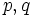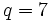# Order has only two prime factors implies prime divisor with larger prime power is core-nontrivial except in finitely many cases

## Name

This result is sometimes termed Burnside's other$p^aq^b$-theorem.

## Statement

Suppose$G$ is a group of order$p^aq^b$, where$p,q$ are distinct primes with$p^a > q^b$ and$a,b$ are positive integers. Then,$p$ is a Core-nontrivial prime divisor (?), except in three kinds of cases. In other words, the$p$-Sylow-core (?) of$G$ is nontrivial; in other words, there is a nontrivial normal$p$-subgroup, except in the following cases:

1.$p = 2$ and$q$ is a Fermat prime (?).
2.$q = 2$ and$p$ is a Mersenne prime (?).
3.$p = 2$ and$q = 7$.

Note that it may also happen that, in addition, the other prime divisor is core-nontrivial.